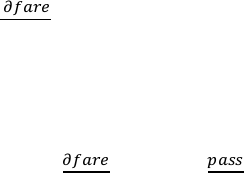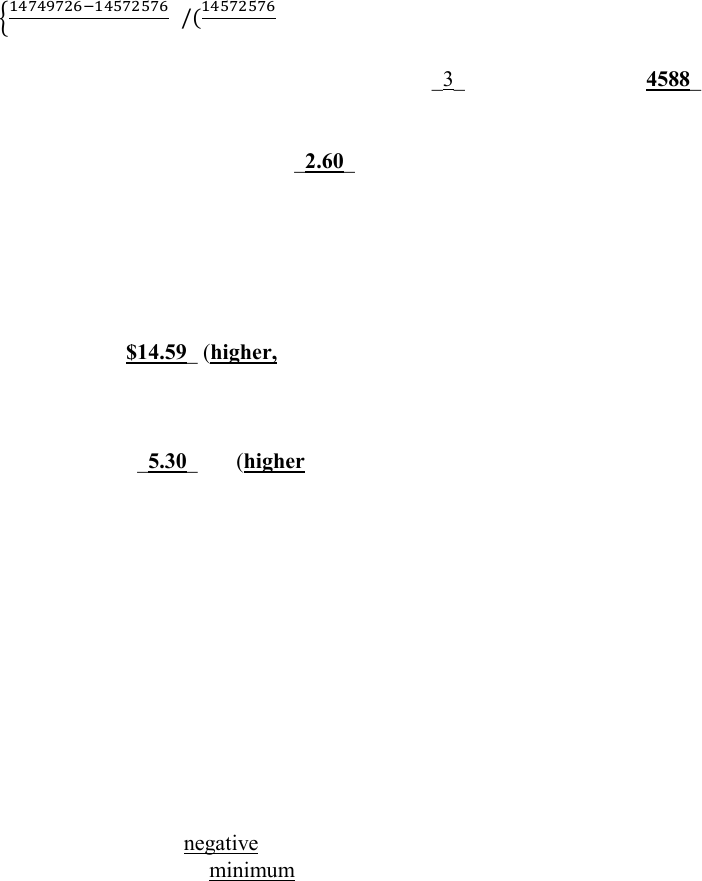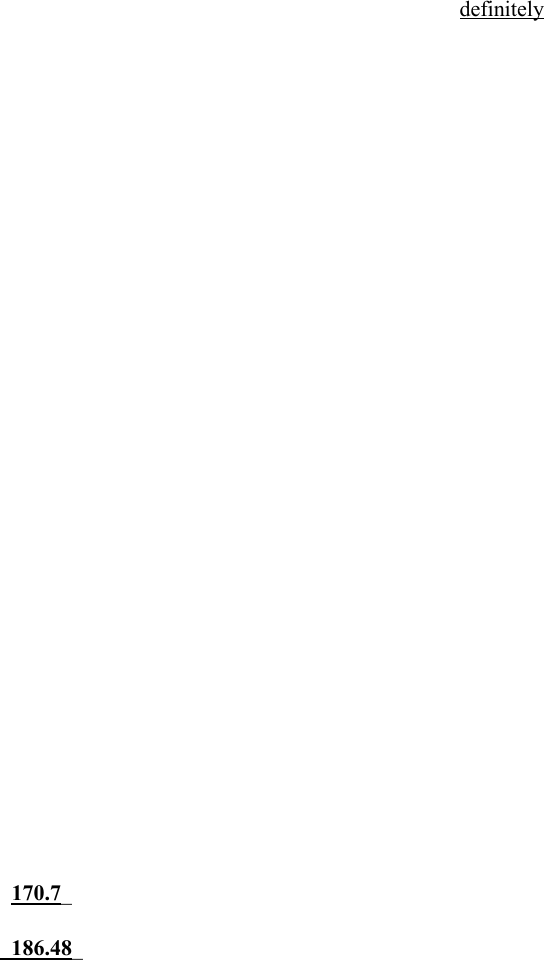# ECO 311 Miami U eco311mt2Fall 1sp17 key with table

19 views11 pages
School
Miami University
Department
Economics
Course
ECO 311
ProfessorEco311, Second Midterm Exam, Spring 2017.
Prof. Bill Even
-1-
ANSWER KEY - FORM 1 (QUESTION 1 IS ON DISTANCE COEFFICIENT)
For the next several questions regarding air-fares, refer to the regression results in table 1.
1. (6 points) Using the first specification, construct a t-statistic for the null hypothesis that distance
has no effect on air-fares.
a. What is the relevant t-statistic?
.0866/.00164=52.80
b. What is the critical level of the t-statistic for rejecting the null hypothesis at the .01 level
(two-tailed test)? [Since the sample size is over 4,000, you may use the standard normal
table for determining the relevant critical value]:
2.57
c. Can you reject the null at the .01 level?
Yes. T-statistic is greater than critical value.
2. Using the first specification, construct a 95% confidence interval for the effect of the distance on
the fare. Show how you derived this confidence interval and circle your answer.
.0866 ±1.96 .00164 = (. , . )
3. Using the first specification, what is the marginal effect of an additional 50 passengers on air fares
if there are currently 100 passengers on the flight? (Recall that the passengers variable and its
square are measured in 100s so passengers/100=1 and its square=1 if passengers=100).
 = 
 (. 5)= [2.227 + 2(.0337)]*.5= -1.08
4. Using the first specification, if the number of passengers can range from 1 to 500
(passengers/100=5), what number of passengers:
a. Maximizes air-fares?
Because 
 < 0 
 =29.53 (2953 ),
    1 
b. Minimizes air-fares? 500 passengers.
Unlock document

This preview shows pages 1-3 of the document.
Unlock all 11 pages and 3 million more documents.Eco311, Second Midterm Exam, Spring 2017.
Prof. Bill Even
-2-
5. Notice that in specification (2), 3 year dummies are added to the first specification.
Based on the information provided in the table, provide an F-statistic for the null hypothesis that the
coefficients on the 3 year dummies are all equal to zero.
a. What is the value of the relevant F-statistic? __________
=
/(
 = 18.59
b. How many degrees of freedom for the numerator? ___3_____ denominator? 4588_
c. What is the critical level for rejection of the null at the .05 level of significance? (A table
with the F-distribution is attached). __2.60__________
d. Can you reject the null that the coefficients on the 3 year dummies are zero at the .0 level of
significance? Yes. F-statistic exceeds critical value.
6. Based on specification (2), other things being the same,
a. Predicted fares are \$14.59_ (higher, lower) in 2000 as compared to 1998. [Provide a \$
value and circle higher/lower.]
b. Predicted fares are \$_5.30____ (higher, lower) in 1999 as compared to 1997.
7. Based on specification (2), if the reference year was changed from 1997 to 2000,
a. What would be the coefficient for the new intercept in the regression?
15.78+54.56= 70.34
b. What would be the coefficient on the 1998 year dummy in the new regression?
1.192-15.78= -14.59
8. (5 points) Suppose that airports in cities with a high cost of living (e.g. Boston, New York, San
Francisco) charge higher airfares. Since there is no control in the regression for cost-of-living
(COL), there could be an omitted variables bias. In two sentences or less, explain how the failure
to control for COL could create a negative bias in the estimated effect of concentration on air-fares
downward. Be sure to indicate the minimum assumptions necessary to assure that the bias is
negative.
The omitted variable bias would be negative if the effect of COL on airfares is positive (as
suggested) and the covariance between COL and concentration is negative. That is, the
coefficient would have a negative bias if cities with a high COL has airports where the market
was less concentrated.
Unlock document

This preview shows pages 1-3 of the document.
Unlock all 11 pages and 3 million more documents.Eco311, Second Midterm Exam, Spring 2017.
Prof. Bill Even
-3-
9. Adding a control for cost-of-living to the air-fares regression will definitely
a. Not reduce R2
c. Increase the sum of squared errors (residuals)
d. All of the above
e. None of the above
10. Adding a control for cost-of-living to the air-fares regressions will cause the standard error on the
estimated coefficient for concentration to:
a. Definitely rise
b. Definitely fall
c. Rise if it has little explanatory power for air fares and is highly correlated with
concentration
d. Fall if it has little explanatory power for air fares and is highly correlated with
concentration
11. Suppose that fares are higher in cities with a high cost of living. Moreover, suppose that cities with
a high cost of living tend to be more populated and therefore have larger flights (i.e. more
passengers). This would cause the estimated effect of passengers on air-fares to have
a. A negative bias
b. A positive bias
c. A negative bias only if the true effect of passengers on air-fares is negative
d. A positive bias only if the true effect of passengers on air-fares is positive
Specification (3) is a regression of air-fares on the same controls as in specification (2), except that all
the controls are measured as “deviations from means” except for the year dummies which are
unchanged.
12. Based on specification (3), what is the predicted air fare for a flight with “average” characteristics
in
a. 1997 170.7________________
b. 2000 _186.48____________
13. Based on specification (3) and remembering that passengers are measured in 100s, what is the
marginal effect of an additional 100 passengers on the air-fare for a flight with average
characteristics but in the year 1997? [Keep in mind that passengers are measured in 100s in the
regression model.]
-1.79
Unlock document

This preview shows pages 1-3 of the document.
Unlock all 11 pages and 3 million more documents.